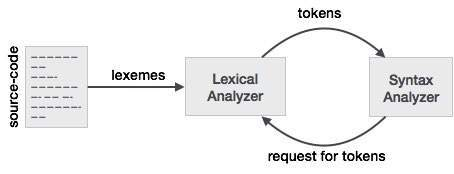Lexical analysis is the first phase of a compiler. It takes the modified source code from language preprocessors that are written in the form of sentences. The lexical analyzer breaks these syntaxes into a series of tokens, by removing any whitespace or comments in the source code.

If the lexical analyzer finds a token invalid, it generates an error. The lexical analyzer works closely with the syntax analyzer. It reads character streams from the source code, checks for legal tokens, and passes the data to the syntax analyzer when it demands.## Tokens

Lexemes are said to be a sequence of characters (alphanumeric) in a token. There are some predefined rules for every lexeme to be identified as a valid token. These rules are defined by grammar rules, by means of a pattern. A pattern explains what can be a token, and these patterns are defined by means of regular expressions.

In programming language, keywords, constants, identifiers, strings, numbers, operators and punctuations symbols can be considered as tokens.

For example, in C language, the variable declaration line

```int value = 100;
```

contains the tokens:

```int (keyword), value (identifier), = (operator), 100 (constant) and ; (symbol).
```

## Specifications of Tokens

Let us understand how the language theory undertakes the following terms:

### Alphabets

Any finite set of symbols {0,1} is a set of binary alphabets, {0,1,2,3,4,5,6,7,8,9,A,B,C,D,E,F} is a set of Hexadecimal alphabets, {a-z, A-Z} is a set of English language alphabets.

### Strings

Any finite sequence of alphabets is called a string. Length of the string is the total number of occurrence of alphabets, e.g., the length of the string tutorialspoint is 14 and is denoted by |tutorialspoint| = 14. A string having no alphabets, i.e. a string of zero length is known as an empty string and is denoted by ε (epsilon).

### Special Symbols

A typical high-level language contains the following symbols:-

 Arithmetic Symbols Addition(+), Subtraction(-), Modulo(%), Multiplication(*), Division(/) Punctuation Comma(,), Semicolon(;), Dot(.), Arrow(->) Assignment = Special Assignment +=, /=, *=, -= Comparison ==, !=, <, <=, >, >= Preprocessor # Location Specifier & Logical &, &&, |, ||, ! Shift Operator >>, >>>, <<, <<<

### Language

A language is considered as a finite set of strings over some finite set of alphabets. Computer languages are considered as finite sets, and mathematically set operations can be performed on them. Finite languages can be described by means of regular expressions.

## Longest Match Rule

When the lexical analyzer read the source-code, it scans the code letter by letter; and when it encounters a whitespace, operator symbol, or special symbols, it decides that a word is completed.

For example:

```int intvalue;
```

While scanning both lexemes till ‘int’, the lexical analyzer cannot determine whether it is a keyword int or the initials of identifier int value.

The Longest Match Rule states that the lexeme scanned should be determined based on the longest match among all the tokens available.

The lexical analyzer also follows rule priority where a reserved word, e.g., a keyword, of a language is given priority over user input. That is, if the lexical analyzer finds a lexeme that matches with any existing reserved word, it should generate an error.